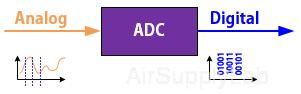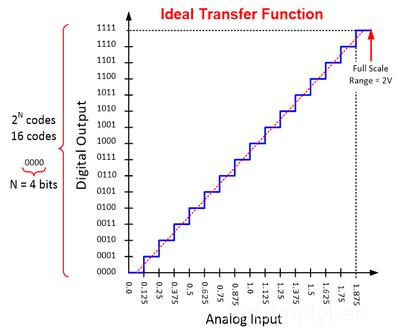# Analog-to-Digital Converter (ADC or A/D Converter)

The signals of the natural environment, such as voice, temperature, light ... and so on, are continuous-time and continuous-amplitude variable (analog) signals. Analog signals have infinite states available. But, the computers we are using are digital signal systems. Digital signals have two states — on (1) or off (0). The analog signals need to be converted into a digital representation which can then be stored in a computer for further processing. An A/D Converter is a device that converts analog signals (usually voltage) obtained from environmental phenomena into digital format. In other words, ADC digitizes an analog signal by converting data with infinite states to a series of pulses. The amplitudes of these pulses can only achieve a finite number of states.Figure 1: Basic Function of ADC

ADCs have the following key characteristics:

1. Input range, or Full-Scale input Range, measured in Volts, is the range of voltages that can be applied at the input of the ADC.
2. Output range, or quantization, measured in bits, is the number of bits in each output sample (typical values are 8, 12, 16, 24, and 32). It is common to say an "8-bit ADC", meaning an ADC with output samples that are 8-bit wide.
3. The sampling rate, measured in Hz, is the rate at which the ADC generates its output samples.

#### Full-Scale Input Range

The full scale input of an ADC is the largest signal amplitude that can be delivered to the converter before the signal is clipped in its digital output representation.

#### Sampling Rate (fs)

The sampling rate is the frequency expressed in Hertz (Hz) at which the ADC samples the input analog signal.

The Nyquist Theorem (or Shannon Theorem) states that an analog signal with a highest frequency of fa MUST be sampled at a sampling rate fs > 2 fa to avoid loss of information.

• If fs < 2 fa then a phenomena called aliasing will occur in the analog signal bandwidth.

#### Resolution

There are two types of resolution in the A/D Conversion: bit-resolution and voltage-resolution.

• The bit-resolution of an ADC refers to the number of bits in the digital output code of the ADC.
• The voltage resolution is the minimum change in input voltage which can be resolved by the ADC. It is the same as analog quantization size.
##### Voltage-Resolution, (or Quantization, Least Significant bit Voltage)

The voltage resolution is represented the minimum change in voltage that is required to guarantee a change in the output code level. It is also called the least significant bit voltage. The quantization of ADC (Q) is equal to the voltage resolution (or the LSB voltage).

The voltage resolution of an ADC is equal to its overall voltage measurement range divided by the total numbers of digital output:
ResolutionVoltageQ = VLSB = (VRef,max - VRef,min) / 2n = VFSR2n    -------- (1)

If you are using an 8-bit ADC, and the voltage input range of the ADC is from 0 to 5 volts. A 8-bit digital value can represent 256 (28 = 256) different numbers. In this example, the bit-resolution of the ADC is 8-bit, and it has a voltage-resolution of 20mV (10v/256).

## The Ideal Transfer Function for A/D Conversion

The transfer function of an ADC is a plot of the voltage input to the ADC versus the code's output by the ADC. The horizontal axis represents a continuous analog input signal. The vertical axis shows the digital output codes which can be thought of as level for rounding off the analog input signal to its nearest digital equivalent. The full scale input range of the ADC is equally divided over the total number of digital output codes to transform the dashed red line to the staircase blue line as shown in Figure 2.Figure 2: Ideal Transfer Function of a 4-bit ADC

The number of bits is the number of binary digits in the digital output code used to represent the full scale analog signal. In this example, we have 4-bit or binary digits ADC, so N = 4, and the number of codes here is 24 = 16, which corresponds to counting from binary 0000 to 111 which is 0 to 15 in decimal, Here, we assume the output of the ADC is linear.

• N: the number of bit ADC
• VFSR: the Full-Scale input Range of the ADC
• VLSB: the minimum resolvable voltage, or ADC voltage resolutionThe full scale input range in this example is 2V (0 to 2 V). The voltage resolution is often identified as the width of the least significant bit, it can be computed by taking the full scale range and dividing by the total number of bits, or 2 to the N power. Therefore, the least significant bit width or voltage resolution can be calculated by dividing 2V by 16 to get a resolution of 0.125V.The full scale range in this example is 2V, but the maximum detectable input voltage is the full scale range minus one LSB or 1.875V in this example.

## Math Conversion Equations

The A/D Converter samples the analog signal and then provides a quantized digital code to represent the input signal. The digital output codes get post-processed and results can be reported to an operator who will use this information to make decisions and take actions. Therefore, it is important to correctly relate the digital codes back to the analog signals it represents.

In general, the ADC input voltage is related to the output code by a simple relationship, as shown in Equation 1:---------- (2)

where Vm (V) is the ADC's input voltage or the measured voltage; Dout  is the ADC's digital-output code in decimal format, and VLSB is the voltage resolution or the value of the least significant bit in the ADC code.

Equation 1 is a general equation that can work for any ADC. It doesn't matter if the ADC's output code is in straight binary or two's complement format, as long as the binary number is correctly converted to its equivalent decimal value.

From here, it is necessary to look at specific ADC architectures in order to determine the best ADC for the job. This includes:

• SAR (Successive Approximation Register) ADCs